# Consider the following statements. (i) The Laplace Transform of 11tet2 cos(et2) is well-defined for some values...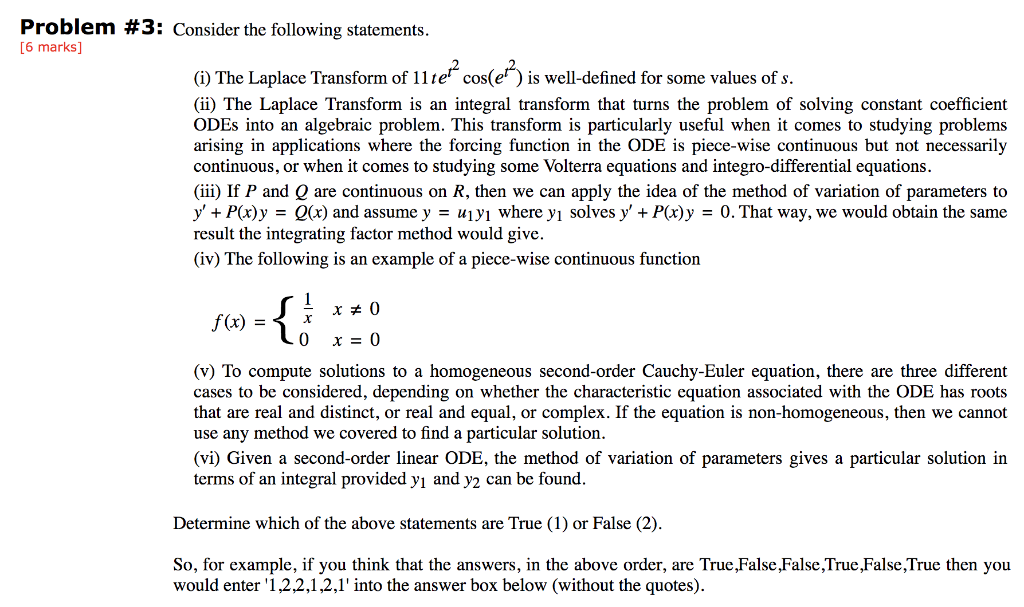Consider the following statements.
(i) The Laplace Transform of 11tet2 cos(et2) is well-defined for some values of s.
(ii) The Laplace Transform is an integral transform that turns the problem of solving constant coefficient ODEs into an algebraic problem. This transform is particularly useful when it comes to studying problems arising in applications where the forcing function in the ODE is piece-wise continuous but not necessarily continuous, or when it comes to studying some Volterra equations and integro-differential equations.
(iii) If P and Q are continuous on R, then we can apply the idea of the method of variation of parameters to y + P(x)y  =  Q(x) and assume y  =  u1y1 where y1 solves y + P(x)y  =  0. That way, we would obtain the same result the integrating factor method would give.
(iv) The following is an example of a piece-wise continuous function

f (x)  =
{
 1 x
x  ≠  0
0 x  =  0
(v) To compute solutions to a homogeneous second-order Cauchy-Euler equation, there are three different cases to be considered, depending on whether the characteristic equation associated with the ODE has roots that are real and distinct, or real and equal, or complex. If the equation is non-homogeneous, then we cannot use any method we covered to find a particular solution.
(vi) Given a second-order linear ODE, the method of variation of parameters gives a particular solution in terms of an integral provided y1 and y2 can be found.

Determine which of the above statements are True (1) or False (2).

So, for example, if you think that the answers, in the above order, are True,False,False,True,False,True then you would enter '1,2,2,1,2,1' into the answer box below (without the quotes).
Problem #3: Consider the following statements. [6 marks] (1) The Laplace Transform of 11 ter cos(e) is well-defined for some values of s. (ii) The Laplace Transform is an integral transform that turns the problem of solving constant coefficient ODEs into an algebraic problem. This transform is particularly useful when it comes to studying problems arising in applications where the forcing function in the ODE is piece-wise continuous but not necessarily continuous, or when it comes to studying some Volterra equations and integro-differential equations. (iii) If P and Q are continuous on R, then we can apply the idea of the method of variation of parameters to y' + P(x)y = Q(x) and assume y = ujyi where yi solves y' + P(x)y = 0. That way, we would obtain the same result the integrating factor method would give. (iv) The following is an example of a piece-wise continuous function x #0 FI* -{ f(x) = 0 x = 0 (v) To compute solutions to a homogeneous second-order Cauchy-Euler equation, there are three different cases to be considered, depending on whether the characteristic equation associated with the ODE has roots that are real and distinct, or real and equal, or complex. If the equation is non-homogeneous, then we cannot use any method we covered to find a particular solution. (vi) Given a second-order linear ODE, the method of variation of parameters gives a particular solution in terms of an integral provided yi and y2 can be found. Determine which of the above statements are True (1) or False (2). So, for example, if you think that the answers, in the above order, are True False, False, True False,True then you would enter '1,2,2,1,2,1' into the answer box below (without the quotes).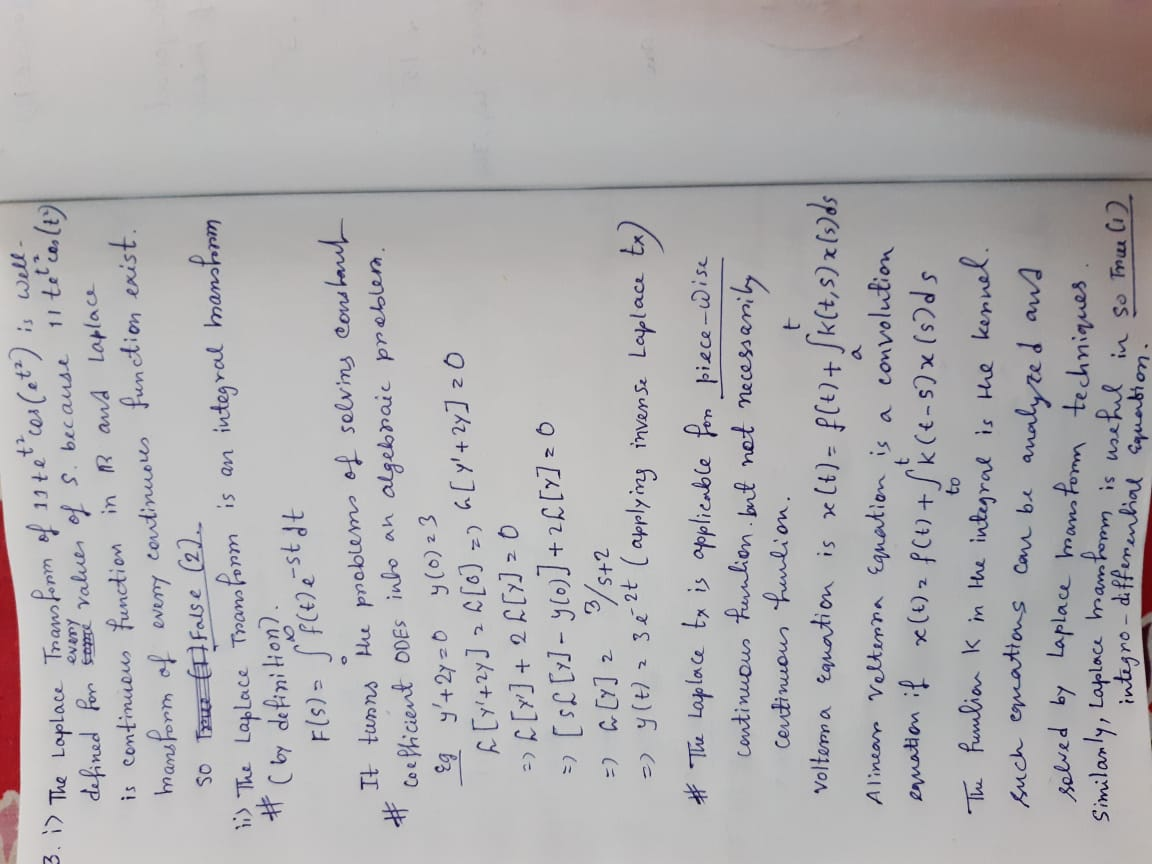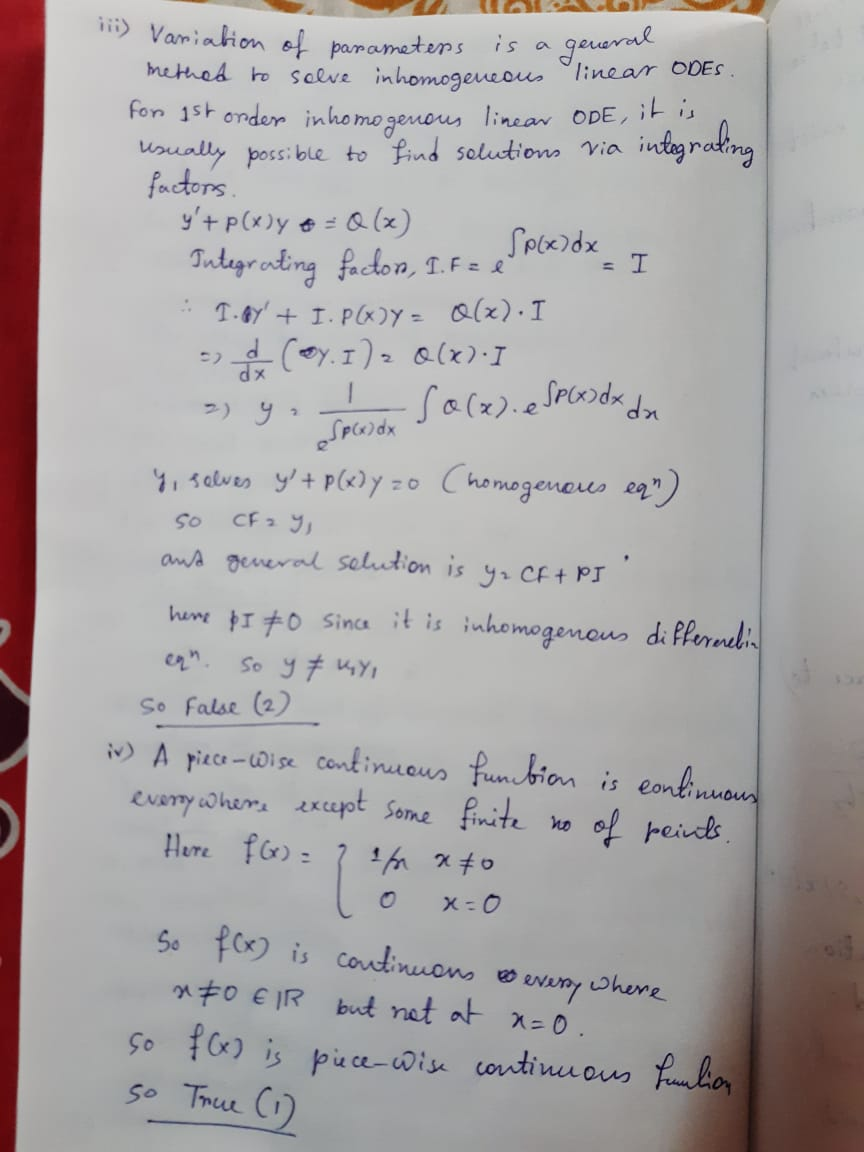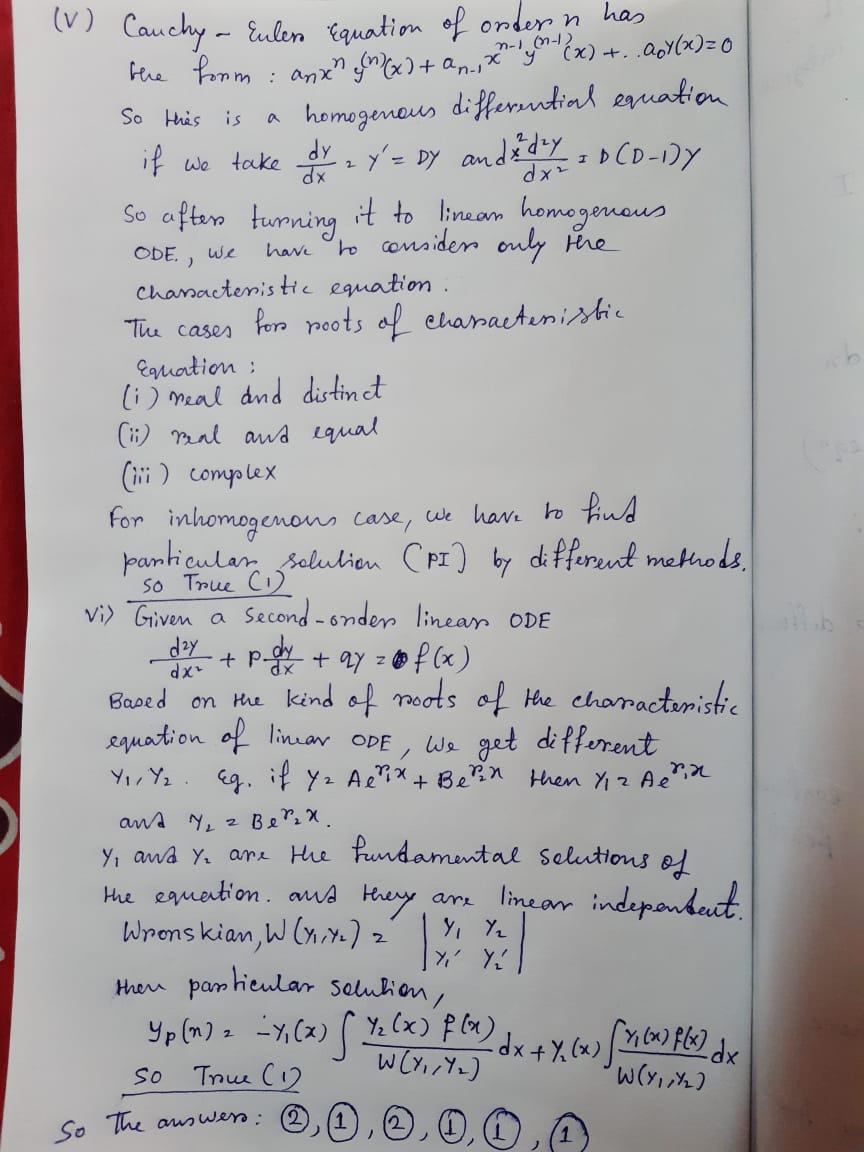##### Add Answer of: Consider the following statements. (i) The Laplace Transform of 11tet2 cos(et2) is well-defined for some values...
Similar Homework Help Questions
• ### Consider the following statements. (i) Given a second-order linear ODE, the method of variation of parameters...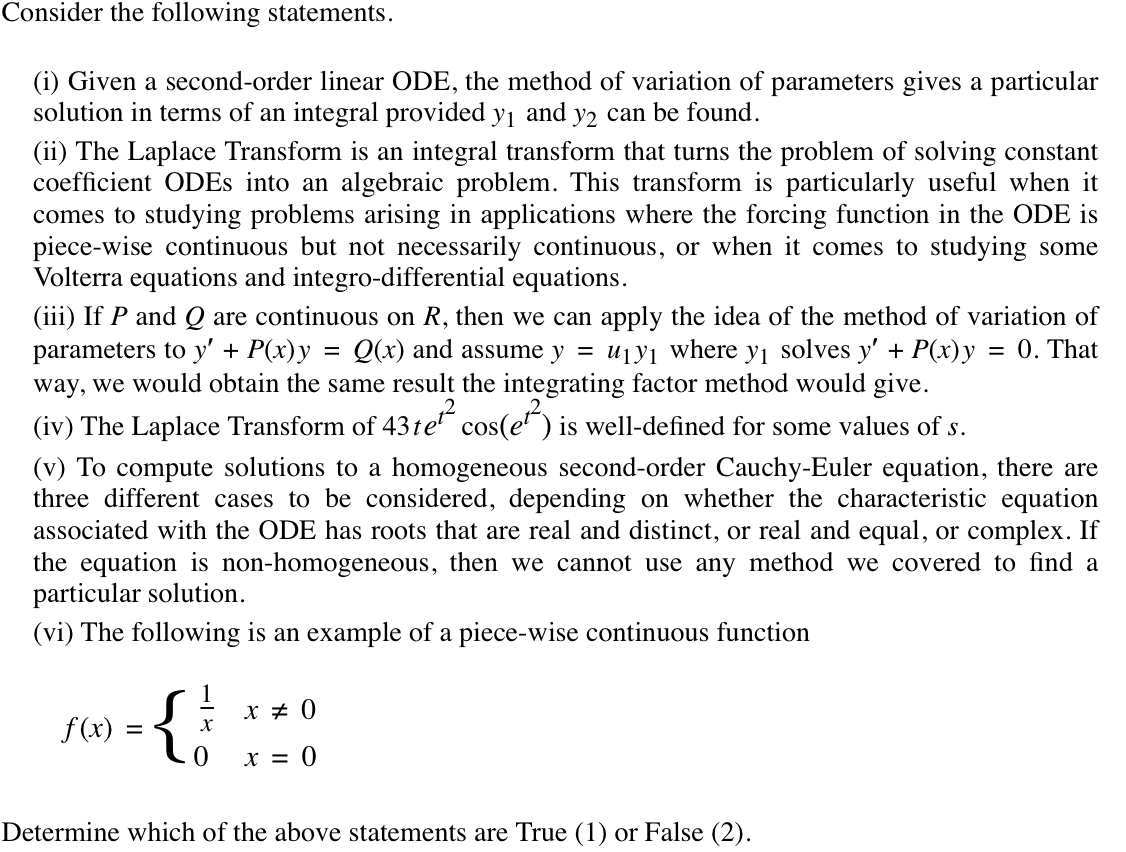Consider the following statements. (i) Given a second-order linear ODE, the method of variation of parameters gives a particular solution in terms of an integral provided y1 and y2 can be found. (ii) The Laplace Transform is an integral transform that turns the problem of solving constant coefficient ODEs into an algebraic problem. This transform is particularly useful when it comes to studying problems arising in applications where the forcing function in the ODE is piece-wise continuous but not necessarily...

• ### Consider the following statements. (i) Spring/mass systems and Series Circuit systems we covered are examples of...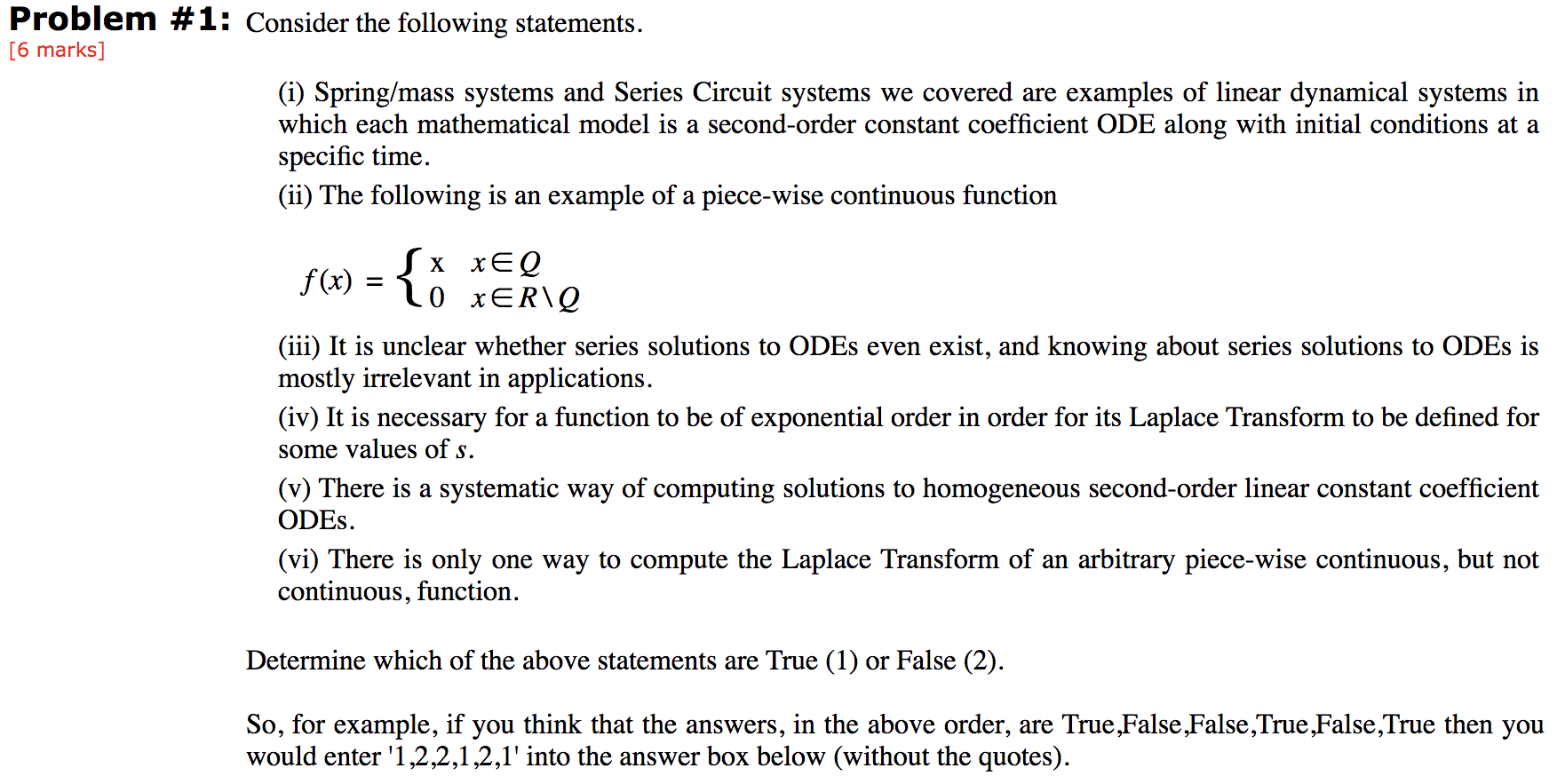Consider the following statements. (i) Spring/mass systems and Series Circuit systems we covered are examples of linear dynamical systems in which each mathematical model is a second-order constant coefficient ODE along with initial conditions at a specific time. (ii) The following is an example of a piece-wise continuous function f (x)  = { x x ∈ Q 0 x ∈ R \ Q (iii) It is unclear whether series solutions to ODEs even exist, and knowing about series solutions to...

• ### Problem #1: Consider the following statements, [6 marks) 6) There is a systematic way of computing...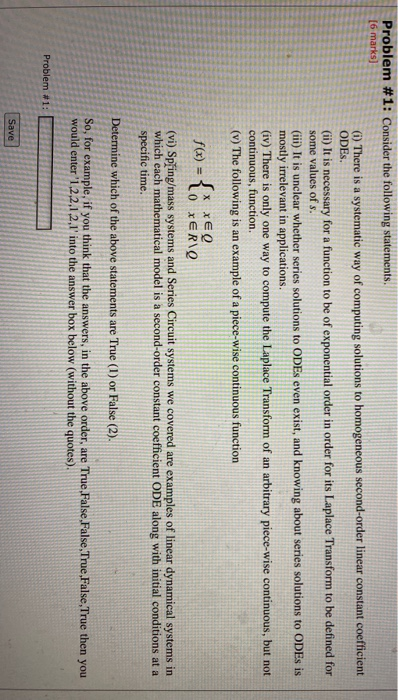Problem #1: Consider the following statements, [6 marks) 6) There is a systematic way of computing solutions to homogeneous second-order linear constant coefficient ODES. (ii) It is necessary for a function to be of exponential order in order for its Laplace Transform to be defined for some values of s. (iii) It is unclear whether series solutions to ODEs even exist, and knowing about series solutions to ODEs is mostly irrelevant in applications. (iv) There is only one way to...

• ### Problem #2: Consider the following statements. [6 marks) (1) The particular solution of the ODE)" -...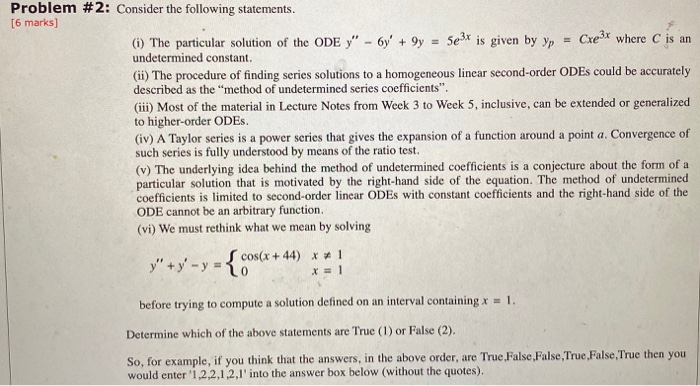Problem #2: Consider the following statements. [6 marks) (1) The particular solution of the ODE)" - 6y' + 9y = 5e3x is given by yp = Cre3x where C is an undetermined constant. (ii) The procedure of finding series solutions to a homogeneous linear second-order ODEs could be accurately described as the "method of undetermined series coefficients". (iii) Most of the material in Lecture Notes from Week 3 to Week 5, inclusive, can be extended or generalized to higher-order ODES...

• ### IGNORE (i) (ii) The procedure of finding series solutions to a homogeneous linear second-order ODEs could...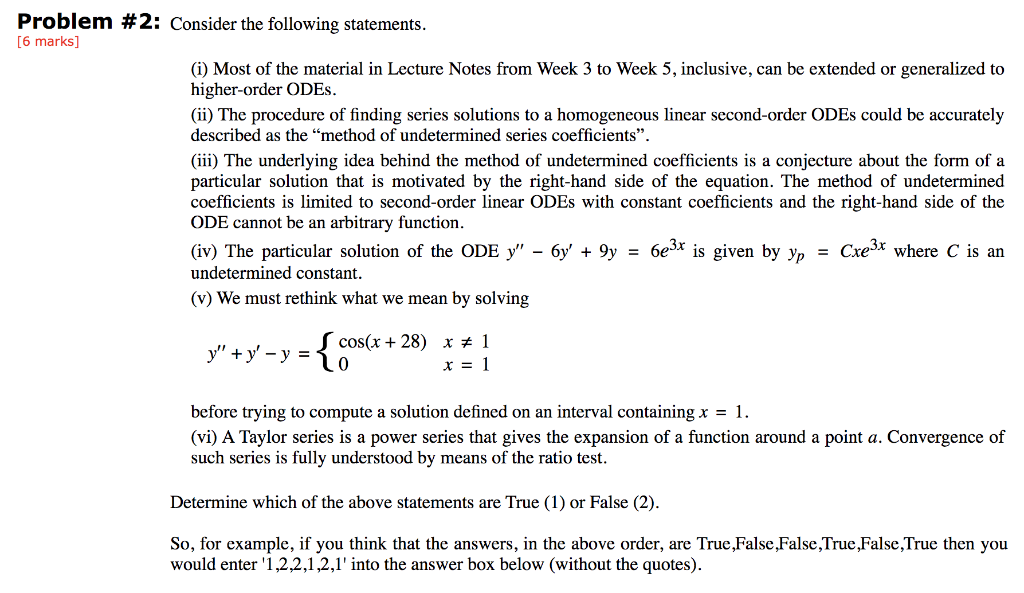IGNORE (i) (ii) The procedure of finding series solutions to a homogeneous linear second-order ODEs could be accurately described as the “method of undetermined series coefficients”. (iii) The underlying idea behind the method of undetermined coefficients is a conjecture about the form of a particular solution that is motivated by the right-hand side of the equation. The method of undetermined coefficients is limited to second-order linear ODEs with constant coefficients and the right-hand side of the ODE cannot be an...

• ### Consider the following statements. (i) A Taylor series is a power series that gives the expansion...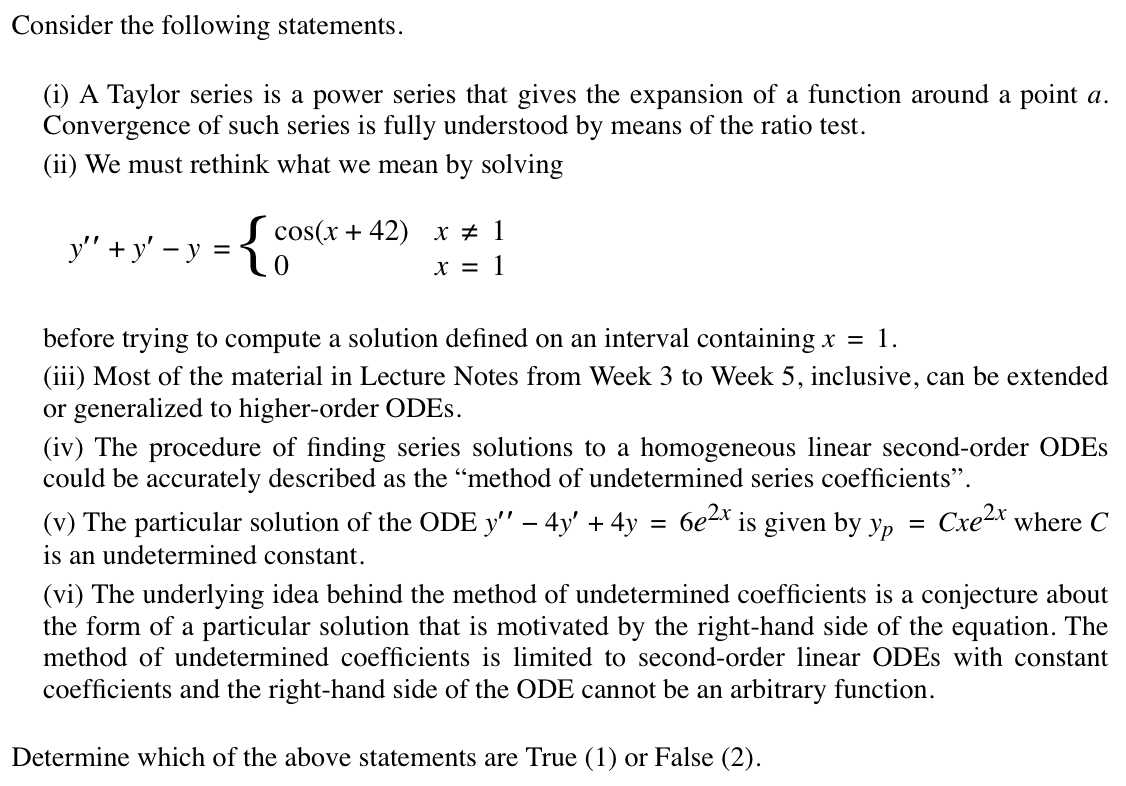Consider the following statements. (i) A Taylor series is a power series that gives the expansion of a function around a point a. Convergence of such series is fully understood by means of the ratio test. (ii) We must rethink what we mean by solving y′′ + y′ − y  =  { cos(x + 42) x  ≠  1 0 x  =  1 before trying to compute a solution defined on an interval containing x  =  1. (iii) Most of the...

• ### please explain the steps as well! it’s imp for me to understand this question. i have attached the table for last part...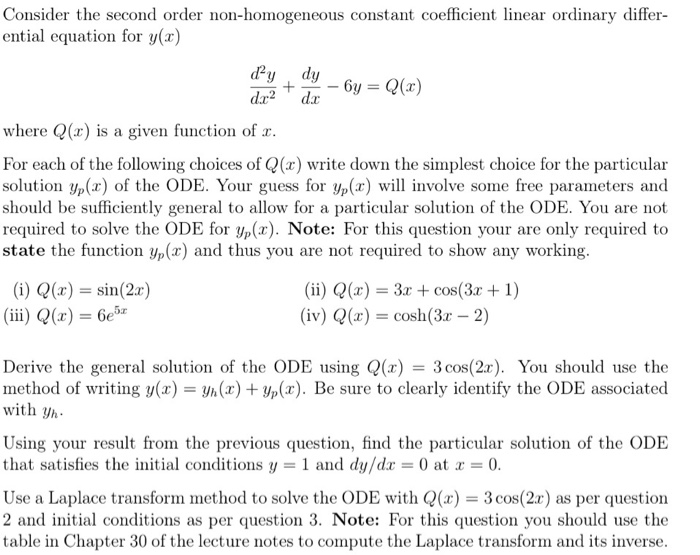please explain the steps as well! it’s imp for me to understand this question. i have attached the table for last part of the question Consider the second order non-homogeneous constant coefficient linear ordinary differ- ential equation for y(x) ору , dy where Q(x) is a given function of r For each of the following choices of Q(x) write down the simplest choice for the particular solution yp(x) of the ODE. Your guess for yp(x) will involve some free parameters...

• ### Engineering Mathematics 1 Page 3 of 10 2. Consider the nonhomogeneous ordinary differential equation ry" 2(r...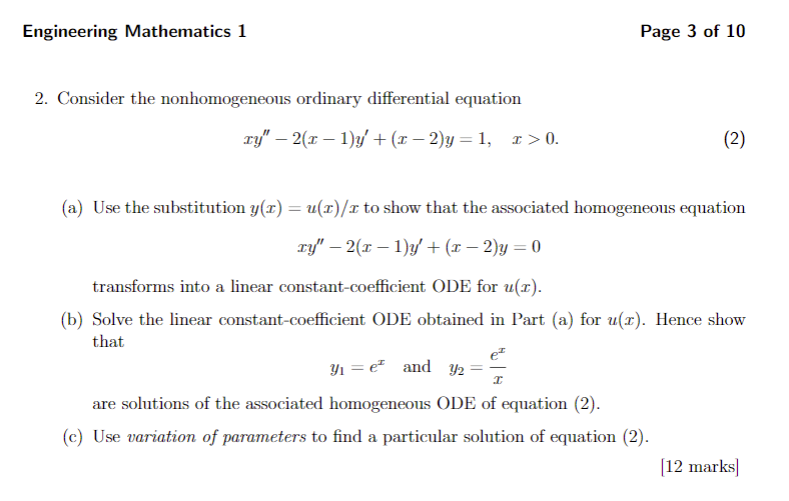Engineering Mathematics 1 Page 3 of 10 2. Consider the nonhomogeneous ordinary differential equation ry" 2(r (x - 2)y 1, (2) r> 0. (a) Use the substitution y(x) = u(x)/x to show that the associated homogeneous equation ry" 2(r (x - 2)y 0 transforms into a linear constant-coefficient ODE for u(r) (b) Solve the linear constant-coefficient ODE obtained in Part (a) for u(x). Hence show that yeand y2= are solutions of the associated homogeneous ODE of equation (2). (c) Use...

• ### (t)= . Use the Laplace transform to solve the following initial value problem: 44" + 2y...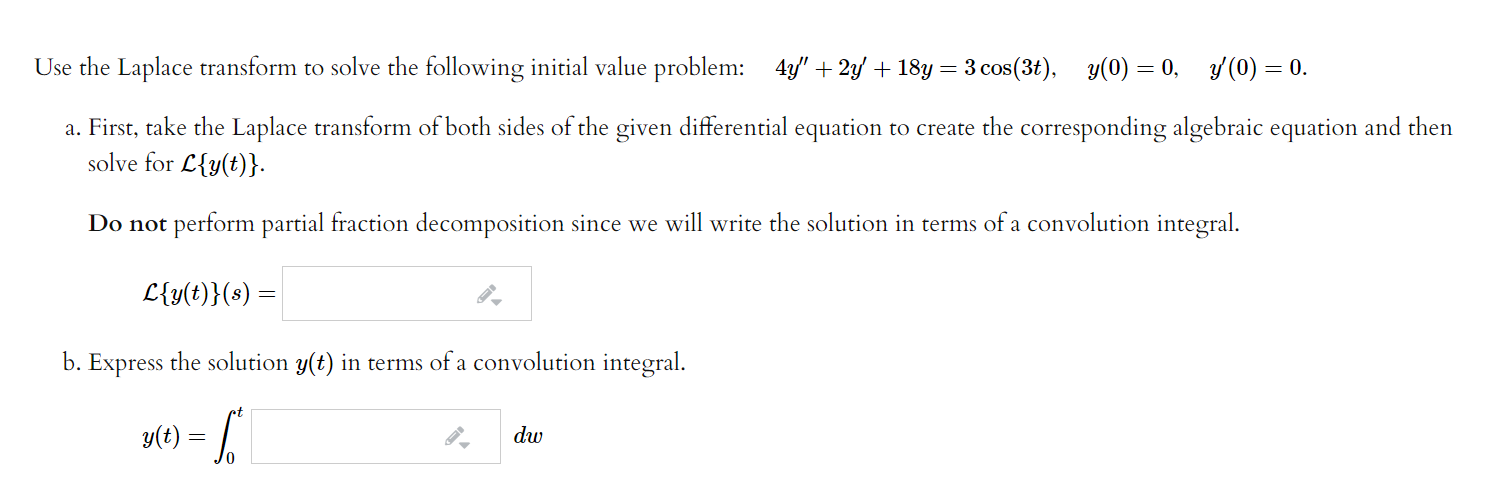(t)= . Use the Laplace transform to solve the following initial value problem: 44" + 2y + 18y = 3 cos(3+), y(0) = 0, y(0) = 0. a. First, take the Laplace transform of both sides of the given differential equation to create the corresponding algebraic equation and then solve for L{y(t)}. Do not perform partial fraction decomposition since we will write the solution in terms of a convolution integral. L{y(t)}(s) b. Express the solution y(t) in terms of a...

• ### Q1] [20 points] In the questions below, circle the correct option (NO DETAILS NECESSARY): i) C{1}...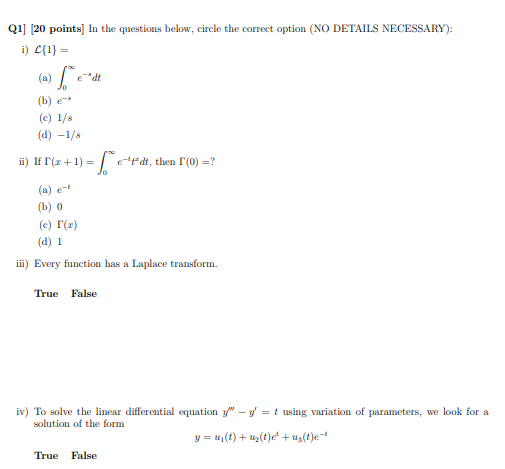Q1] [20 points] In the questions below, circle the correct option (NO DETAILS NECESSARY): i) C{1} = (a) - [*ed (b) e (c) 1/8 (d) -1/8 ii) If (x + 1) = e-Pdt, then (0) =? (a) et (b) 0 (c) 1 (1) (d) 1 iii) Every function has a Laplace transform. True False iv) To solve the linear differential equation y" - y = t using variation of parameters, we look for a solution of the form y =...

Free Homework App